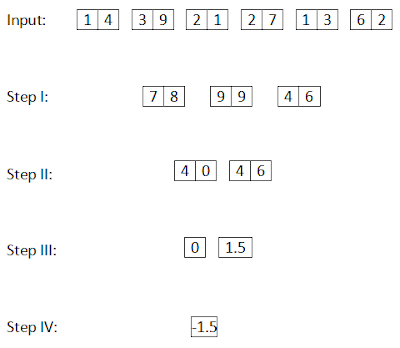## Reasoning Input Output based on SBI PO Mains | IBPS | RBI | NICL

Reasoning Input Output based on SBI PO Mains
Directions (1 – 5): Study the following information carefully and answer the given questions.
A number arrangement machine arranges two digit numbers in typical manner. The first step has been obtained by multiplying the digits in input. Multiplication has not been done in any other steps. They are obtained by applying certain logic. Each step is a resultant of previous step.1. If each middle digit of all the three numbers in the step I are halved and then added, then what will be the final sum?
A) 4
B) 6.5
C) 3.5
D) 7.5
E) None of these
2. If the final output is subtracted from ‘5’, then what will be the resultant value?
A) -2.8
B) -3.2
C) 2.8
D) 3.2
E) None of these
3. Which of the following combination represent the first digit of the third number and second digit of the first number in step I of the given input?
A) (5,2)
B) (8,3)
C) (3,8)
D) (2,5)
E) (2,4)
4. Which of the following represent the difference between the third digit of the third number and second digit of the first number in step I?
A) 4
B) 5
C) 6
D) -4
E) None of these
5. What is the multiplication of three numbers obtained in step II?
A) 100
B) 120
C) 240
D) 220
E) None of these
Directions (6 – 10): Study the following information carefully and answer the given questions.
A number arrangement machine arranges two digit numbers in typical manner. The first step has been obtained by multiplying the digits in input. Multiplication has not been done in any other steps. They are obtained by applying certain logic. Each step is a resultant of previous step.6. If each digit in the step II is halved and then added, then what will be the final sum?
A) 4
B) 7
C) 3.5
D) 8
E) None of these
7. If the value ‘7.5’ is subtracted from the final output, then what will be the resultant value?
A) 6
B) 8
C) -9
D) -6
E) None of these
8. Which of the following combination represent the first digit of the third number and second digit of the first number in step I of the given input?
A) (8,4)
B) (7,4)
C) (8,6)
D) (4,8)
E) (4,7)
9. Which of the following represent the difference between the first digit of the second number and second digit of the first number in step II?
A) 4
B) 0
C) 6
D) -4
E) None of these
10. What is the multiplication of two numbers obtained in step III?
A) 6.0
B) 1.6
C) 2.0
D) 0
E) None of these
Solutions:
(1 – 5):In step I: 7* 5 = 35; 3*2 = 6;
5* 8 = 40; 6*1 = 6;
4* 7 = 28; 9*1 = 9;
In step II: each first digit and third digit of the numbers are added and then subtracted with second digit.
(6 + 3) - 5 = 4; (6 + 4) - 0 = 10; (2+9) – 8 = 3;
In step III: divide the second number by the first number and also third number by second number.
10/4 = 2.5; 3/10=0.3;
In step IV: subtract the second number from first number. This is the final output.
2.5– 0.3 = 2.2
1. B) 5/2 + 0/2 + 8/2 = 2.5 + 0 + 4= 6.5
2. C)
3. D) The first digit of the third number in step I is ‘2’ and the second digit of the first number is ‘5’. Hence, (2, 5)
4. A) The third digit of the third number in step I is ‘9’ and second digit of the first number in step I is ‘5’. Hence, 9 – 5 =4
5. B) The two numbers obtained in step II are 4, 10 and 3. Hence, 4 *10*3 = 120
(6 – 10):In step I: 1* 7 = 7; 4*2 = 8;
3* 3 = 9; 9*1 = 9;
2* 2 = 4; 1*6 = 6;
In step II: each first digits of three numbers are added and multiplied by two and each second digits also do same.
7 + 9 + 4 = 20* 2 = 40; 8 + 9 + 6 = 23*2 = 46;
In step III: divide the second digit by first digit in each two numbers.
0/4 = 0; 6/4=1.5;
In step IV: subtract the second number from first number. This is the final output.
0 – 1.5 = -1.5
6. B) 4/2 + 0/2 + 4/2 + 6/2 = 2 + 0 + 2 + 3 = 7
7. C) The final output is ‘ -1.5’ = -1.5 – 7.5 = - 9.0
8. D) The first digit of the third number in step I is ‘4’ and the second digit of the first number is ‘8’. Hence, (4, 8)
9. A) The first digit of the second number in step II is ‘4’ and second digit of the first number in step II is ‘0’. Hence, 4 – 0 =4
10. D) The two numbers obtained in step III are 0 and 1.5. Hence, 0 * 1.5 = 0